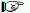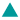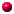VHDL Tutorial: Learn by Example
-- by Weijun Zhang, July 2001*** NEW (2010): See the new book VHDL for Digital Design, F. Vahid and R. Lysecky, J. Wiley and Sons, 2007. Concise (180 pages), numerous examples, low-cost. Also see www.ddvahid.com.***

If we hear, we forget; if we see, we remember; if we do, we understand.
--  Proverb
ESD book | Dalton Project | VHDL Reference | Synopsys Tutorial | ActiveHDL Tutorial | Xilinx TutorialBasic Logic GatesCombinational Logic DesignTypical Combinatinal Logic ComponentsLatch and Flip-FlopsSequential Logic DesignTypical Sequential Logic ComponentsCustom Single-Purpose Processor DesignGeneral-Purpose Processor Design Appendix: Modeling an industry coreForeword (by Frank Vahid)

<> HDL (Hardware Description Language) based design has established itself as the modern approach to design of digital systems, with VHDL (VHSIC Hardware Description Language) and Verilog HDL being the two dominant HDLs. Numerous universities thus introduce their students to VHDL (or Verilog). The problem is that VHDL is complex due to its generality. Introducing students to the language first, and then showing them how to design digital systems with the language, tends to confuse students. The language issues tend to distract them from the understanding of digital components. And the synthesis subset issues of the language add to the confusion.

We developed the following tutorial based on the philosophy that the beginning student need not understand the details of VHDL -- instead, they should be able to modify examples to build the desired basic circuits. Thus, they learn the importance of HDL-based digital design, without having to learn the complexities of HDLs. Those complexities can be reserved for a second, more advanced course. The examples are mostly from the textbook Embedded System Design by Frank Vahid and Tony Givargis. They start from basic gates and work their way up to a simple microprocessor. Most of the examples have been simulated by Aldec ActiveHDL Simulator and Synopsys Design Analyzer, as well as synthesized with Synopsys Design Compiler . Several sequential design examples have been successfully tested on Xilinx Foundation Software and FPGA/CPLD board.Basic Logic Gates
(ESD Chapter 2: Figure 2.3)
Every VHDL design description consists of at least one entity / architecture pair, or one entity with multiple architectures. The entity section of the HDL design is used to declare the I/O ports of the circuit, while the description code resides within architecture portion. Standardized design libraries are typically used and are included prior to the entity declaration. This is accomplished by including the code "library ieee;" and "use ieee.std_logic_1164.all;".
 Driver Behavior Code Behavior Simulation Inverter Behavior Code Behavior Simulation OR gate Behavior Code Behavior Simulation NOR gate Behavior Code Behavior Simulation AND gate Behavior Code Behavior Simulation NAND gate Behavior Code Behavior Simulation XOR gate Behavior Code Behavior Simulation XNOR gate Behavior Code Behavior SimulationCombinational Logic Design
(ESD Chapter 2: Figure 2.4)
We use port map statement to achieve the structural model (components instantiations). The following example shows how to write the program to incorporate multiple components in the design of a more complex circuit. In order to simulate the design, a simple test bench code must be written to apply a sequence of inputs (Stimulators) to the circuit being tested (UUT). The output of the test bench and UUT interaction can be observed in the simulation waveform window.
 Combinational Logic Behavior Code Test Bench Behavior Simulation Synthesis Schematic Gate-level Simulation Tri-State Driver Behavior Code Test Bench Behavior Simulation Synthesis Schematic Gate-level SimulationDiscussion I: Signal vs. Variable

Siganls are used to connect the design components and must carry the information between current statements of the design. On the other hand, variables are used within process to compute certain values. The following example shows their difference:

 Signal/Variable Example Behavior Code Behavior SimulationTypical Combinational Components
(ESD Chapter 2: Figure 2.5)
The following behavior style codes demonstrate the concurrent and sequential capabilities of VHDL. The concurrent statements are written within the body of an architecture. They include concurrent signal assignment, concurrent process and component instantiations (port map statement). Sequential statements are written within a process statement, function or procedure. Sequential statement include case statement, if-then-else statement and loop statement.
 Multiplexor Behavior Code Test Bench Behavior Simulation Synthesis Schematic Gate-level Simulation Decoder Behavior Code Test Bench Behavior Simulation Synthesis Schematic Gate-level Simulation Adder Behavior Code Test Bench Behavior Simulation Synthesis Schematic Gate-level Simulation Comparator Behavior Code Test Bench Behavior Simulation Synthesis Schematic Gate-level Simulation ALU Behavior Code Test Bench Behavior Simulation Synthesis Schematic Gate-level Simulation Multiplier Behavior Code Test Bench Behavior Simulation Synthesis Schematic Gate-level SimulationLatch & Flip-Flops
(ESD Chapter 2.3)
Besides from the circuit input and output signals, there are normally two other important signals, reset and clock, in the sequential circuit. The reset signal is either active-high or active-low status and the circuit status transition can occur at either clock rising-edge or falling-edge. Flip-Flop is a basic component of the sequential circuits.
 Simple Latch Behavior Code Test Bench Behvaior Simulation Gate-level Implementation Gate-level Simulation D Flip-Flop Behavior Code Test Bench Behavior Simulation Gate-level Implementation Gate-level Simulation JK Flip-Flop Behavior Code Test Bench Behavior Simulation Gate-level Implementation Gate-level SimulationTypical Sequential Components
(ESD Chapter 2: Figure 2.6)
Typical sequential components consist of registers, shifters and counters. The concept of generics is often used to parameterize these components. Parameterized components make it possible to construct standardized libraries of shared models. In the behavioral description, the output transitions are generally set at the clock rising-edge. This is accomplished with the combination of the VHDL conditional statements (clock'event and clock='1'). During the testbench running, the expected output of the circuit is compared with the results of simulation to verify the circuit design.
 Register Behavior Code Test Bench Behavior Simulation Gate-level Implementation Synthesis Schematic Structural Simulation Shift Register Behavior Code Test Bench Behavior Simulation Gate-level Implementation Synthesis Schematic Structural Simulation Counter Behavior Code Test Bench Behavior Simulation Gate-level Implementation Synthesis Schematic Structural SimulationSequential Logic Design
(ESD Chapter 2: Figure 2.7)
The most important description model presented here may be the Finite State Machine (FSM). A general model of a FSM consists of both the combinational Logic and sequential components such as state registers, which record the states of circuit and are updated synchronously on the rising edge of the clock signal. The output function computes the various outputs according to different states. Another type of sequential model is the memory module, which usually takes a long time to be synthesized due to the number of design cells.
 FSM Model Behavior Code Test Bench Behavior Simulation Gate-level Implementation Synthesis Schematic Gate-level Simulation
• Memories (ESD Chapter 5)
 RAM Module Behavior Code Test Bench Behavior Simulation Gate-level Implementation Synthesis Schematic Gate-level Simulation ROM Module Behavior Code Test Bench Behavior Simulation Gate-level Implementation Synthesis Schematic Gate-level SimulationDiscussion II: Behavior vs. RTL Synthesis (Y Chart)

RTL stands for Register-Transfer Level. It is an essential part of top-down digital design process. Logic synthesis offers an automated route from an RTL design to a Gate-Level design. In RTL design a circuit is described as a set of registers and a set of transfer functions describing the flow of data between the registers, (ie. FSM + Datapath). As an important part of a complex design, this division is the main objective of the hardware designer using synthesis. The Synopsys Synthesis Example illustrates that the RTL synthesis is more efficient than the behavior synthesis, although the simulation of previous one requires a few clock cycles.

 GCD Caculator Behavior Code RTL Code (FSM+D) Comparison

Following section illustrates the RTL (FSM+Datapath) method further using several design examples.Custom Single-Purpose Processor Design
(ESD Chapter 2, Chapter 4)
The first three examples illustrate the difference between RTL FSMD model (Finite State Machine with Datapath buildin) and RTL FSM + DataPath model. From view of RT level design, each digital design consists of a Control Unit (FSM) and a Datapath. The datapath consists of storage units such as registers and memories, and combinational units such as ALUs, adders, multipliers, shifters, and comparators. The datapath takes the operands from storage units, performs the computation in the combinatorial units, and returns the results to the storage units during each state. This process typically takes one or two clock cycles.

Data-flow (looks more like an Algorithm) modeling is presented in the fourth example. The FIR digital filter algorithm is simulated and synthesized using VHDL. A comparison of the coding styles between the RTL modeling and Algorithm level modeling highlights the different techniques.

• GCD Calculator (ESD Chapter2: Figure 2.9-2.11)
 FSMD Modeling RTL Code Test Bench RTL Code Simulation Gate-level Implementation Synthesis Schematic Gate-level Simulation FSM + Datapath Modeling RTL Code Test Bench RTL Code Simulation Gate-level Implementation Synthesis Schematic Gate-level Simulation
• Simple Bridge (ESD Chapter 2: Figure 2.13-2.14)
 FSMD Modeling RTL Code Test Bench RTL Code Simulation Gate-level Implementation Synthesis Schematic Gate-level Simulation FSM + Datapath Modeling RTL Code Test Bench RTL Code Simulation Gate-level Implementation Synthesis Schematic Gate-level Simulation
• ISA Bus Interface (ESD Chapter 4, Chapter 6)
 FSM + Datapath Modeling RTL Code Test Bench RTL Code Simulation Gate-level Implementation Synthesis Schematic Gate-level Simulation
• FIR Digital Filter (DSP Example)
 Data-Flow Modeling Behavior Code Test Bench Behavior Simulation(1,2) Gate-level Implementation Synthesis Schematic Gate-level SimulationDiscussion III: Synopsys Power Analysis

Synopsys tools can be used to perform Power Analysis for all the VHDL designs. Generally, the better design has smaller power consumption. On the other hand, improve the power always means sacrificing other design metrics such as performance, area size or NRE cost. Therefore, a designer need to balance these metrics to find the best implementation for the given application and constraints. Please check out the power analysis results of Adder, Counter, ISA controller, Bridge controller and FIR Filter. As we expected, FIR digital filter has the biggest power consumption because it has a more complex circuit doing DSP computation. Synopsys power analysis tutorial can be found here.Discussion IV: Synthesis with Timing Constraints

When we design and simulate the high-level (either behavior or RTL) code, we only care about design functionality. However, in VHDL synthesis, the timing and the functionality of a design must always be considered together. Therefore, once the design has been synthesized, the second goal of simulation is to quickly verify that the gate-level implementation meets timing requirements. We use this idea (coding -> simulation -> synthesis -> simulation) to test all of the examples in this tutorial.

Another common way is to apply the timing constrains on the design during synthesis. then the timing report is checked to see if the slack, which is the required delay minus the actual delay, is MET or VIOLATED. If VIOLATED, we should go back to the VHDL code and re-write it to improve timing. The whole design will be compiled and tested again.

 Counter Behavior Code Sythesis Script File Timing ReportDiscussion V: Relationship between Area and Timing

During Synopsys synthesis, ordinary combinational logic will go through several of what are known as mapping optimizations. In a normal optimization, the synthesis tool will optimize in relation to the set constrains. It is usual to talk about moving along a "banana curve" on the area and time axes. This means that the tougher the timing constrains, the larger the design will be, and vice versa. The results from two different synthesis constrains applied on the same design are shown below.

 FIR filter Sample Synthesis Script Comparison Table Banana CurveGeneral-Purpose Processor Design
(ESD Book Chapter 3, Figure 3.15)
As indicated in the previous part, an Application Specific Integrated Circuit (ASIC) is specified with behavior descriptions which are presented in the form of particular algorithm or flowchart. A general purpose processor, on the other hand, is specified completely by its instruction set (IS). A sequence of instructions is required for the computation of a mathematical expression or any other similar computational task. To illustrate the whole procedure, a simple Pseudo-Microprocessor model is used which contains seven instructions (ESD book figure 3.7). The RT level design method from previous examples is used again to construct this microprocessor. The CPU will fetch, decode, and execute each instruction in order to get the final result.

For test purposes, a short program (sequential instructions) is loaded into the memory. After execution, this program will obtain 10  Fabonacci Numbers, and store the results into specific memory address. The design was implemented using Active-HDL and Synopsys Design Compiler. (Please note that PC.vhd need a little modify to get correct synthesis result. Just a practice for the reader.)

 Top Level Structural Code microprocessor.vhd Design Hierarchy cpuhierarchy.jpg Test Bench of CPU Design TB_mp.vhd Control Unit ctrl_unit.vhd Block Diagram cpublock.jpg Data Path datapath.vhd controller.vhd  PC.vhd IR.vhd   bigmux.vhd Memory memory.vhd smallmux.vhd reg_file.vhd alu.vhd obuf.vhd
 Synopsys Sythesis Script Files cpusyn.scr syn_ctrl_unit.inc, syn_PC.inc, syn_IR.inc, syn_datapath.inc, syn_reg_file.inc syn_alu.inc, syn_memory.inc syn_controller.inc, syn_obuf.inc, syn_bigmux.inc, syn_smallmux.inc
 Behavor Simulation  Script File cpusim.scr Simulation Waveform cpusim1.jpg cpusim2.jpg Gate-Level Simulation  Script File cpugatesim.scrDiscussion V: VHDL vs. Verilog

There are now two industry standard hardware description languages, VHDL and Verilog. It is important that a designer knows both of them although we are using only VHDL in class. Verilog is easier to understand and use. For several years it has been the language of choice for industrial applications that required both simulation and synthesis. It lacks, however, constructs needed for system level specifications. VHDL is more complex, thus difficult to learn and use. However it offers a lot more flexibility of the coding styles and is suitable for handling very complex designs. Here is a great article to explain their difference and tradeoffs.Appendix: Modeling a real industry chip - HD 6402
(ESD Chapter 4)

I. Specification of HD 6402

II. Behavior Modeling of UART Transmitter
(1) Behavior Code (2) Gate-level design (3) Test Benches - 1, 2, 3 (4) Synopsys Simulation
Case#1: one 8-bit word, 1 start, 2 stops, and even parity, or Data=11000101, Control Word=11011.  ( Gate-level Simulation )
Case#2: three 5-bit words, 1 start, 1 stop, and no parity, or Data=11010 & 00101 & 10001, Control Word=00100.  ( Gate-level Simulation )
Case#3: two 6-bit words, 1 start, 2 stops, and odd parity, or Data=110010 & 101101, Control Word=01000.  ( Gate-level Simulation )

III. Behavior Modeling of UART Receiver
(1) Behavior Code (2) Gate-level design (3) Test Benches - 1, 2, 3 (4) Synopsys Simulation
Case#1: two 6-bit words, 1 start, 2 stops, and even parity, (Data=111001 & 100101, Control Word=01101). ( Gate-level Design Simulation )
Case#2: one 8-bit words, 1 start, 1 stop, and odd parity, (Data=10111001, Control Word=11000). ( Gate-level Design Simulation )
Case#3: three 5-bit words, 1 start, 1 stop, and no parity, (Data=01001 & 01110 & 00100, Control Word=00010. ( Gate-level Design Simulation )

IV. Structural Modeling of HD-6402
(1) Behavior Code (2) Gate-level design (3) Test Bench (4) Synopsys Simulation

Created by Weijun Zhang (weijun_92507@yahoo.com)
at UC, Riverside, 06/2001